﻿ Infinite Energy Explains the Continues Behavior of Electrostatic Phenomenon Between ParticlesEnergy and Power

p-ISSN: 2163-159X    e-ISSN: 2163-1603

2020;  10(2): 27-30

doi:10.5923/j.ep.20201002.01### Infinite Energy Explains the Continues Behavior of Electrostatic Phenomenon Between Particles

Yehya Amari

USTHB, Algeria

Correspondence to: Yehya Amari, USTHB, Algeria.
 Email: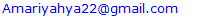Abstract

What is known as banding energy is in fact the energy needed to separate atoms, the real banding energy is match more than this; it is the energy that holds atoms together despite the permanent vibration. This work is in continuity with previous ones that predict the same thing. This work is an analysis that ends with a proposal as a solution for the problem of energy and it sources. Characteristics of the electron (Size and Speed ...) were placed in the law of magnetic field; this led to one of most verified laws of electricity, which is the Coulomb low. Basing on this result, another real parameter which is relativity of the physical quantities was introduced by the replacement of what led to the Coulomb force. All this brought us to the conclusion that justifies the behavior of the mater, it is the existence of the Infinite Energy.

Keywords: Infinite Energy, Clean Energy, Bonding Energy

Cite this paper: Yehya Amari, Infinite Energy Explains the Continues Behavior of Electrostatic Phenomenon Between Particles, Energy and Power, Vol. 10 No. 2, 2020, pp. 27-30. doi: 10.5923/j.ep.20201002.01.

### 1. Introduction

This work came to make a simplified continuity with the work of G. Moscariello et al. detailed in . The phenomenon that historically lead to think about electricity and further the behavior of matter, is the force that attracts light bodies (dust, paper,…) to other electrically charged bodies, it is the Coulomb force  The question that arises in order to understand the source of this attraction from an energetic point of view is: What is the real phenomenon that results this attraction?. “The lift the paper and dust needs energy because each have a mass”.
Saying that the electrostatic is the origin of this force is not a complete answer in our research, many works such as   and  speaks about coulomb energy. However, from the definition of energy as a physical quantity and the way this phenomenon is happening, we can find a contradiction between the way this phenomenon happens and the quantities of energy described in.
Then view the law of the electrostatic force that is not dependent on time but only three factors that will limit the amount of dust that will attach to the another charged body which are: the amount of charges, the distance and the weight. This force appears every time when the conditions are available . Using an energy vision to analyze this phenomenon, we take the example where dust is attracted to a glass stick:
To lift dust, the needed amount of energy is: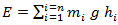(1)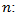The number of dust seeds.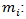The mass of each dust seed.Gravity.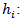The distance between the bar and the position of each seed of dust.
Imagine that this dust will be eliminated just when he arrives at the glass stick, while for the manner by which this phenomenon occurs, another dust will take its place and so follows even if this experiment is repeated for an infinite number of times, and this is verified theoretically and experimentally.
This force that pulls dust toward the glass stick is a force responsible for many phenomena that surround us, it is the force that holds atoms together in chemical molecules . So far. Deepen the analysis of the experience of attracting dust to the glass bar. To give a justification with a convincing depth that allows us to have an idea about the origin of this energy responsible of moving the body.
Following the experiences that satisfy the laws of electromagnetism , optoelectronics , thermodynamics , electrodynamics   and quantum mechanics  it is clear that an electromagnetic cloud is part of the constitution of the electron.
The directions of the electrostatic forces are perpendicular to the flow of electromagnetic cloud.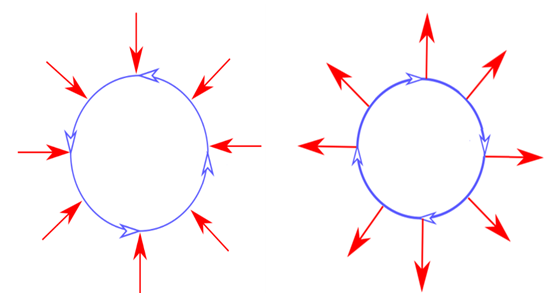Figure 1. Forces perpendicular to the flow of the EM wave

### 2. Analysis Using the Lorentz Force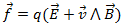(2)
From the expression of the Lorentz force, a sequence of magnetic and electric phenomena can be predicted as follows : By the presence of a charge under the influence of an electric field E, there is an emergence of an electric force which will give acceleration to this charge. The movement of this charge will give rise to a magnetic force and the presence of a magnetic field.
This reading leads us to another question, and also to see the law from another angle and see the other possibilities that it can reveal other properties of the electromagnetic phenomenon. This law seems like it is the addition of two separable phenomena as follows: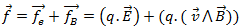(3)
which presents this equation (3) is that it is possible at least to have a magnetic force without the presence of electrical field E, that is to say, with E = 0: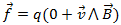(4)
This gives us an expression for the Laplace force. Applying the final analysis on the latter equation with a mathematical reading translated to a physical phenomenon, is found that without the movement of the charge, the magnetic force cannot take place, then the occurrence of a magnetic force always requires moving charges.

### 3. An Analysis Using Maxwell Equations

The content of this analysis is explained more clearly by the Maxwell equations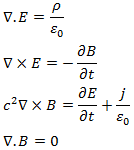Taking these equations to their static state means that there is no charge variation in function of time and all charges are in their static and motionless. These equations will become :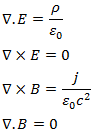Looking at these equations, it is found that they are separable into two distinct types from each other. They are electrostatic and magnetism. But a magnetic phenomenon also exists in these circumstances The non-existence of a movement in space which translates to speed, which in turn why: B ≠ 0. In reality, this stability is in our reference space. But what justifies this result is that the charge turns on itself in a closed-circuit which is shown clearly by the first magnetic equation: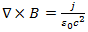which shows that without this current in a stable closed circuit in this reference space (does not depend on time of a macro vision) this equation will be: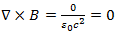The problem lies in the reading of this conclusion, saying that they are independent of each other doesn’t mean that each can be found single and isolated from the other, taking into account the time factor which is always present, their concept becomes clearer, they never were found separated in nature. However, in reality proven by experience, the electric force depends on the charge movement because there is no experience that was able to study a charge in its purely static state, this amounts to the impossibility of excluding the time factor at this level because it becomes an uncontrollable appearance. Taking the experimental results of the force of a charge, it is seen that the force decreases with increasing distance between the charges which means decreases with the decrease of the electrostatic field. More exactly and follows a field theory: the reduction of the field is the decrease of the influence of the physical quantity generated by the source of this quantity on influenced by this phenomenon. To understand what is really happening to give the later three points in order to provide a solid explanation for these phenomena, basing on the results of experiments, in this topic and using the deductions of the composition of particles follows certain characteristics they exhibit. Basing on the previous example and make an analogy to make an analysis on the magnetic phenomenon that accompanies this movement, basing this analysis on the basic equations governing this movement. It is known that the magnetic momentum of an atom resulting current loops of electrons and spins.

### 4. Electrostatic Force from Magnetism

Taking one of the fundamental laws of the force acting on an electric charge moving particle known by the Lorentz law known by: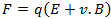Taking an electron with a charge which turns around itself, and then studying the magnetic force with which it acts on a charge q that is following this law this force will be given by: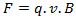To clarify the concept, by the return to a well-known phenomenon in the field of physics that is the spin known in classical mechanics as the angular momentum of rotation. For our study, it is enough for us that this is limited to the very basic definition that states that the spin is a rotation of the particle around itself, this phenomenon for the electron creates a field of a physical quantity corresponding to a torque. This very fundamental definition is sufficient to give us proof of ownership to exploit this possibility for use in the field of renewable energy. It is very well known experimentally that the value of the magnetic field B is proportional to the current by a constant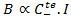it is also known that this constant is of value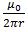then the value of the magnetic field that accompanies the circulation of an electric current can be written in the following form: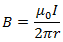Applying the latter on a spherical electric charge that revolves around itself: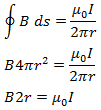It is known that: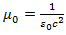So: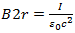For the current of this charge that it revolves around itself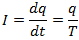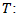The period of each turn.
So the value of T is from the speed that is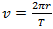and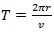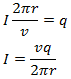The equation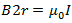becomes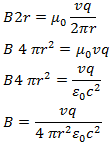If we take that this charge is an electron. To replace the speed: For an electron, the radius is equal to: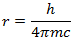and Zitterbewegung frequency  is equal to: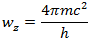Then the speed will be: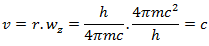By replacing on B: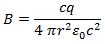If this electron is interacting with another, automatically it has the same speed then: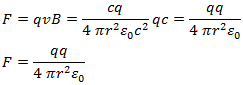Following this analysis, it is found that the magnetic force is the origin of the so-called electrostatic force.
By using the two concepts known by magnetism and electricity and the combination with an example of the classical mechanics one will go out by one a simplification which helps to better understand what is going on. It has been previously seen that the force on a load under the influence of another moving charge is composed of two forces which together constitute the Lorentz force .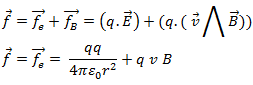This is analogous to a very simple example in physics.

### 5. Relativistic Analysis

Looking at this phenomenon with an energy-vision, we will analyse this problem using a relative vision given by relativity  by taking:
• The electron that turns around itself “as shown above, by the speed of light c”.
• The scalar nature of energy.
To explain another aspect that accompanies this phenomenon. The results will be different and energy formula will be: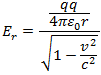And as shown previously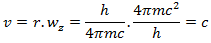So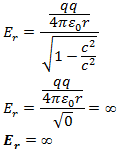The potential energy of an electronic charge relative to another is INFINITE what changes it is the trace of the existence or the operation of this remarkable energy by the value of the force applied due to the distance between the charges. This energy appears every time a charged particle enters into the field of another charged particle, despite the number of times. These results explain this behaviour. There were many works on this topic for many years, there are even contemporary works such as  and  but this one is more simple and direct. If we look to the binding energy such as described in  and  and many other works we find that they actually talk about the needed energy to separate molecules and atoms from each other, but as long as molecules and atoms are linked to each other, there is this energy to make them like they are despite the time and physical constraints.

### 6. Conclusions

The potential energy of an electronic charge relative to another is INFINITE what changes it is the trace of the existence or the operation of this remarkable energy by the value of the force applied due to the distance between the charges. All this represents the answers to the questions and hypotheses put at the beginning, what keeps matter in permanent assembly despite the factor of time and the destabilizing phenomena is this infinite energy, it also provides us a convincing reason for the continuation of the Attraction of the dust towards the glass bar. This result can be very useful for improving the quality of human life, protection of the environment and the balance of the ecosystem and the preservation of sources of natural wealth for future generations.

### References

  C. A. COULOMB, "Ou l'on d~termine suivant quelles lois le fluide magn6tique ainsi que le fluide ~lectrique agissent soit par repulsion, soit par attraction," M~m. de l'Acad. Sci., 1785; "Collection de M~m.," Paris, I, p. 116. (1884).  E. H. Auerbach, S. Kahana, and J. Weneser CALCULATION OF THE COULOMB ENERGY SHIFT BETWEEN Sc41 AND Ca41. PHYSICAL REVIEW LETTERS. VOLUME 23, NUMBER 21. (1969).  M.A. Bentleya, S.M. Lenzi, Coulomb energy differences between high-spin states in isobaric multiplets, Progress in Particle and Nuclear Physics 59, 497–561, (2007).  P. L. A. Popelier and D. S. Kosov, Atom–atom partitioning of intramolecular and intermolecular Coulomb energy, JOURNAL OF CHEMICAL PHYSICS VOLUME 114, NUMBER 15 (2001).  B.Delley, D. E. Ellis, and A. J. Freeman, Binding energy and electronic structure of small copper particles, PHYSICAL REVIEw B VOLUME 27, NUMBER 4, (1983).  LEYSEP T. B, SpectralS tructureo f StimulatedE lectromagnetEicm issions Between Electron Cyclotron Harmonics, JOURNAL OF GEOPHYSICAL RESEARCH, VOL. 98, NO. AI0, PAGES 17, 597-17, 606, (1993).  O. Schmidt et al., Time-resolved two photon photoemission electron microscopy, Applied Physics B, 223–227 (2002).  A. P. Ramirez et al., Thermodynamic and Electron Diffraction Signatures of Charge and Spin Ordering in La12xCaxMnO3, PHY S I CAL REV I EW LETTERS, VOLUME 76, NUMBER 17, (1996).  M. Skrzypek and S. Jadach, Exact and approximate solutions for the electron nonsinglet structure function in QED, Z. Phys. C - Particles and Fields 49, 577 584 (1991).  LARRYK E., Solvated Electron Structure in Glassy Matrices, Acc. Chem. Res., 14, 138-145, (1981).  V. Popsueva et al., Structure of lateral two-electron quantum dot molecules in electromagnetic fields, PHYSICAL REVIEW B 76, 035303 (2007).  B. A. Kemp, T. M. Grzegorczyk, and J. A. Kong, LORENTZ FORCE ON DIELECTRIC AND MAGNETIC PARTICLES, J. of Electromagn. Waves and Appl., Vol. 20, No. 6, 827–839, (2006).  J. C. MAXWELL, "Treatise on Electricity and Magnetism," Third edition, Oxford, Clarendon Press, 1892, Chap. 23.  Richard P. Feynman. The Feynman LECTURES ON PHYSICS, Volume II: mainly Electromagnetism end matter, chapter 4, 6, 7, 13.  J. Maruani, The Dirac Electron: From Quantum Chemistry to Holistic Cosmology, JOURNAL OF THE CHINESE CHEMICAL SOCIETY, Volume 63, 33-48 (2016).  K. JOHNSON, Quantum Electrodynamics in the Infinite Energy Limit, ANNALS OF PHYSICS: 10, 536-552 (1960).  G. Moscariello, A. Passarelli di Napoli and M. M. Porzio, Existence of Infinite Energy Solutions of Degenerate Elliptic Equations, Journal for Analysis and its Applications Volume 31, 393–426, (2012).  B. Delley, D. E. Ellis, and A. J. Freeman, Binding energy and electronic structure of small copper particles, PHYSICAL REVIEW VOLUME 27, NUMBER 4, (1983).  B. Johansson, Core-level binding-energy shifts for the metallic elements, PHYSICAL REVIEW B VOLUME 21, (1980).